# Excel DATEVALUE Function

This post will guide you how to use Excel DATEVALUE function with syntax and examples in Microsoft excel.

### Description

The Excel DATEVALUE function returns the serial number of date. And it can be used to convert a date represented as text format into a serial number that recognizes as a date format.

For example, you can convert a date “2018/03/04” that is text format to a serial number, you can use the formula: =DATEVALUE (“2018/03/04”). Then it returns a serial number “43163” you can use the Excel dates to manipulate with formulas or pivot table to filter, sort. So it is very useful than the text dates.

The DATEVALUE function is a build-in function in Microsoft Excel and it is categorized as a DATE and TIME Function.

The DATEVALUE function is available in Excel 2016, Excel 2013, Excel 2010, Excel 2007, Excel 2003, Excel XP, Excel 2000, Excel 2011 for Mac.

### Syntax

The syntax of the DATEVALUE function is as below:

`=DATEVALUE(date_text)`

Where the EDATE function arguments is:

• Date_text -This is a required argument. A valid date that the format is text. Or the text that represents a date in an excel date format.

Note:

• The date_text argument must represent a date between January 1, 1900 and December 31, 9999. The DATEVALUE function returns the #VALUE! Error value if the value of the date_text argument falls outside of this range.
• If the year portion of the date_text argument is omitted, the DATEVALUE function uses the current year from your computer’s built-in clock. Time information in the date_text argument is ignored.
• January 1 1900 is serial number 1 by default.

### Excel DATEVALUE Function Examples

The below examples will show you how to use Excel DATEVALUE Function to return a serial number of a given date.

1# Get a serial number of a date (03/14/2018) the format as text, enter the following formula in Cell B1.

`=DATEVALUE("03/14/2018")`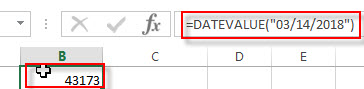2# Get a serial number of a date (14-May-2018) the format as text, enter the following formula in Cell B2.

`=DATEVALUE("14-May-2018")`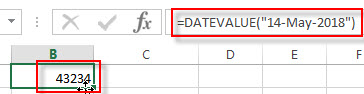3# Get a serial number of a date (2018/03/14) the format as text, enter the following formula in Cell B3.

`=DATEVALUE("2018/03/14")`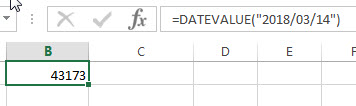4# get a serial number from a date that the year portion is omitted (03/04) assuming the current year is 2018, using the following formula:

`=DATEVALUE("03/14")`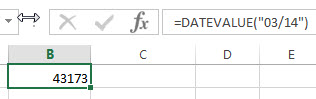5# get a serial number from a date that created by combining the values in Cells A1, A2, and A3

`=DATEVALUE(A1&"/"&A2&"/"&A3)`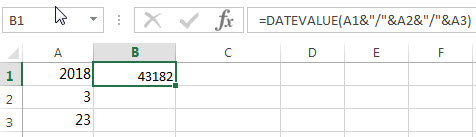### Related Functions

• Excel DAY function
The Excel DAY function returns a day of a date (from 1 to 31). The DAY function is a build-in function in Microsoft Excel and it is categorized as a DATE and TIME Function.The syntax of the DAY function is as below:= DAY (date_value)
• Excel YEAR function
The Excel YEAR function returns a four-digit year from a given date value, the year is returned as an integer ranging from 1900 to 9999. The syntax of the YEAR function is as below:=YEAR (serial_number)…
• Excel MONTH function
The Excel MONTH function returns the month of a date represented by a serial number. And the month is an integer number from 1 to 12. The syntax of the MONTH function is as below:=MONTH (serial_number)…

### More Excel Datevalue Function Examples

• Convert Month Name to Number
If you want to convert month name to number with an excel formula, or you want to convert 3 letter month name to numbers, you can create a formual based on the MONTH function and the DATEVALUE function…
• Compare Dates
Assuming that you have a list of data that contain date values in Excel, you can use the IF function to create a formula to achieve it. If the date is greater that the given date value, then return True. Otherwise, it returns False….